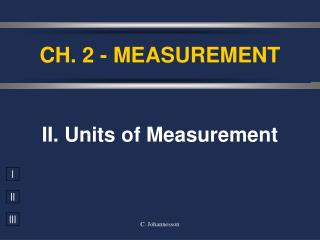DownloadDownload PresentationCH. 2 - MEASUREMENT

# CH. 2 - MEASUREMENT

Download Presentation## CH. 2 - MEASUREMENT

- - - - - - - - - - - - - - - - - - - - - - - - - - - E N D - - - - - - - - - - - - - - - - - - - - - - - - - - -
##### Presentation Transcript

1. CH. 2 - MEASUREMENT II. Units of Measurement C. Johannesson

2. A. Number vs. Quantity • Quantity - number + unit C. Johannesson UNITS MATTER!!

3. B. SI Units Quantity Symbol Base Unit Abbrev. Length l meter m Mass m kilogram kg Time t second s Temp T kelvin K Amount n mole mol C. Johannesson

4. kilo- mega- M k 103 106 deci- BASE UNIT d --- 100 10-1 centi- c 10-2 milli- m 10-3 micro-  10-6 nano- n 10-9 pico- p 10-12 B. SI Units Prefix Symbol Factor C. Johannesson

5. M V D = C. Derived Units • Combination of base units. • Volume (m3 or cm3) • length  length  length 1 cm3 = 1 mL 1 dm3 = 1 L • Density (kg/m3 or g/cm3) • mass per volume C. Johannesson

6. D. Density Mass (g) Volume (cm3) C. Johannesson

7. Problem-Solving Steps 1. Analyze 2. Plan 3. Compute 4. Evaluate C. Johannesson

8. D. Density • An object has a volume of 825 cm3 and a density of 13.6 g/cm3. Find its mass. GIVEN: V = 825 cm3 D = 13.6 g/cm3 M = ? WORK: M = DV M = (13.6 g/cm3)(825cm3) M = 11,200 g C. Johannesson

9. WORK: V = M D V = 25 g 0.87 g/mL D. Density • A liquid has a density of 0.87 g/mL. What volume is occupied by 25 g of the liquid? GIVEN: D = 0.87 g/mL V = ? M = 25 g V = 29 mL C. Johannesson